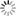# Episode List

OR

### Season 1

1 Oct. 1968
The BonusKnow what this is about?
Be the first one to add a plot.

8 Oct. 1968
The Fireplace FirmKnow what this is about?
Be the first one to add a plot.

15 Oct. 1968
You Can Only Buy OnceKnow what this is about?
Be the first one to add a plot.

22 Oct. 1968
The Last of the Big SpendersKnow what this is about?
Be the first one to add a plot.

29 Oct. 1968
Money for ChangeKnow what this is about?
Be the first one to add a plot.

5 Nov. 1968
Of Course We Trust You ArnoldKnow what this is about?
Be the first one to add a plot.

12 Nov. 1968
West of EdenKnow what this is about?
Be the first one to add a plot.

19 Nov. 1968
The Right Attitude?Know what this is about?
Be the first one to add a plot.

Season 1  Season 2  »

## Contribute to This PageAnswer